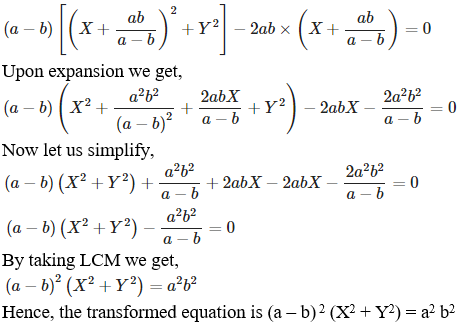• +91 9971497814
• info@interviewmaterial.com

# RD Chapter 22- Brief Review of Cartesian System of Rectangular Coordinates Ex-22.3 Interview Questions Answers

### Related Subjects

Question 1 :

What does theequation (x – a) 2 + (y – b) 2 = r2 becomewhen the axes are transferred to parallel axes through the point (a-c, b)?

Given:

The equation, (x – a) 2 +(y – b) 2 = r2

The given equation (x – a)2 +(y – b)2 = r2 can be transformed into the newequation by changing x by x – a + c and y by y – b, i.e. substitution of x by x+ a and y by y + b.

((x + a – c) – a)2 +((y – b ) – b)2 = r2

(x – c)2 + y2 = r2

x2 + c2 – 2cx + y2 =r2

x2 + y2 -2cx = r2 –c2

Hence, the transformed equation is x2 +y2 -2cx = r2 – c2

Question 2 :

What does theequation (a – b) (x2 + y2) – 2abx = 0 become if the origin isshifted to the point (ab / (a-b), 0) without rotation?

Given:

The equation (a – b) (x2 +y2) – 2abx = 0

The given equation (a – b) (x2 +y2) – 2abx = 0 can be transformedinto new equation by changing x by [X + ab / (a-b)] and y by YQuestion 3 :

Find what thefollowing equations become when the origin is shifted to the point (1, 1)?
(i) x2 +xy – 3x – y + 2 = 0
(ii) x2 –y2 – 2x + 2y = 0
(iii) xy – x – y + 1 = 0
(iv) xy – y2 –x + y = 0

(i) x2 + xy – 3x – y + 2 = 0

Firstly let us substitute the value of x by x + 1 and y by y + 1

Then,

(x + 1)2 + (x +1) (y + 1) – 3(x + 1) – (y + 1) + 2 = 0

x2 + 1 + 2x + xy+ x + y + 1 – 3x – 3 – y – 1 + 2 = 0

Upon simplification we get,

x2 + xy = 0

Thetransformed equation is x2 + xy= 0.

(ii) x2 –y2 – 2x + 2y = 0

Let us substitute the value of x by x + 1 and y by y + 1

Then,

(x + 1)2 – (y + 1)2 – 2(x + 1) + 2(y + 1) = 0

x2 + 1 + 2x – y2 – 1 – 2y – 2x – 2 + 2y + 2 = 0

Upon simplification we get,

x2 – y2 = 0

Thetransformed equation is x2 – y2 = 0.

(iii) xy – x – y + 1 = 0

Let us substitute the value of x by x + 1 and y by y + 1

Then,

(x + 1) (y + 1) – (x + 1) – (y + 1) + 1 = 0

xy + x + y + 1 – x – 1 – y – 1 + 1 = 0

Upon simplification we get,

xy = 0

Thetransformed equation is xy = 0.

(iv) xy – y2 –x + y = 0

Let us substitute the value of x by x + 1 and y by y + 1

Then,

(x + 1) (y + 1) – (y + 1)2 –(x + 1) + (y + 1) = 0

xy + x + y + 1 – y2 –1 – 2y – x – 1 + y + 1 = 0

Upon simplification we get,

xy – y2 = 0

Thetransformed equation is xy – y2 =0.

4. At what point the origin beshifted so that the equation x2 +xy – 3x + 2 = 0 does not contain any first-degree term and constant term?

Solution:

Given:

The equation x2 +xy – 3x + 2 = 0

We know that the origin has been shifted from (0, 0) to (p, q)

So any arbitrary point (x, y) will also be converted as (x + p,y + q).

The new equation is:

(x + p)2 + (x +p)(y + q) – 3(x + p) + 2 = 0

Upon simplification,

x2 + p2 + 2px + xy + py + qx + pq – 3x – 3p+ 2 = 0

x2 + xy + x(2p +q – 3) + y(q – 1) + p2 + pq – 3p– q + 2 = 0

For no first degree term, we have 2p + q – 3 = 0 and p – 1 = 0,and

For no constant term we have p2 +pq – 3p – q + 2 = 0.

By solving these simultaneous equations we have p = 1 and q = 1from first equation.

The values p = 1 and q = 1 satisfies p2 +pq – 3p – q + 2 = 0.

Hence, the point to which origin must be shifted is (p, q) = (1,1).

Question 4 : Verify that the area of the triangle with vertices (2, 3), (5, 7) and (-3 -1) remains invariant under the translation of axes when the origin is shifted to the point (-1, 3).

Given:

The points (2, 3), (5, 7), and (-3, -1).

The area of triangle with vertices (x1, y1),(x2, y2), and (x3, y3) is

= ½ [x1(y2 – y3) + x2(y3 -y1)+ x3(y1 – y2)]

The area of given triangle = ½ [2(7+1) + 5(-1-3) – 3(3-7)]

= ½ [16 – 20 + 12]

= ½ 

= 4

Origin shifted to point (-1, 3), the new coordinates of thetriangle are (3, 0), (6, 4), and (-2, -4) obtained from subtracting a point(-1, 3).

The new area of triangle = ½ [3(4-(-4)) + 6(-4-0) – 2(0-4)]

= ½ [24-24+8]

= ½ 

= 4

Since the area of the triangle before and after thetranslation after shifting of origin remains same, i.e. 4.

We cansay that the area of a triangle is invariant to shifting of origin.

Todays Deals### RD Chapter 22- Brief Review of Cartesian System of Rectangular Coordinates Ex-22.3 Contributorskrishan

Name:
Email:

# Latest News# 9000 interview questions in different categories